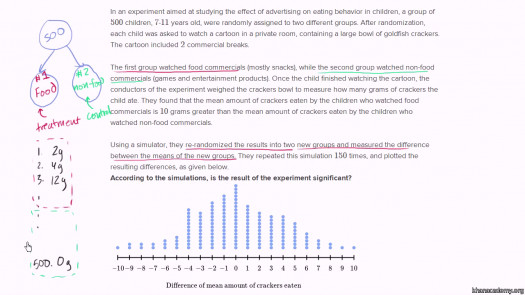# Difference Between Groups Using Two-sample Inference

10 Questions | Total Attempts: 110SettingsWith a combination of significance tests and confidence intervals, evaluate your knowledge of how to use two-sample inference to define the difference between groups.

• 1.
Hypotheses convening the relative size of the means of two population are tested by...
• A.

Sample value and P-value

• B.

Critical Values and P-value

• C.

Confidence Interval

• D.

Point estimate and P-value

• 2.
Independent values are composed of groups that are in no way matched to them.
• A.

Always true

• B.

Often true

• C.

Occasionally true

• D.

Never true

• 3.
A point estimate for the difference in two group population is the...
• A.

Difference in different sample means

• B.

Difference in standardised test statistics

• C.

Similarities in different sample means

• D.

Difference in corresponding sample means

• 4.
A dependent sample is one where two groups are formed from matched pairs.
• A.

Always true

• B.

Often true

• C.

Occasionally true

• D.

Never true

• 5.
Which of these is the procedure used to test hypotheses concerning a single population?
• A.

3 steps

• B.

4 steps

• C.

5 steps

• D.

6 steps

• 6.
The null and alternative hypotheses will always be expressed in terms of...
• A.

Similarities of the two population means

• B.

Difference in confidence interval

• C.

Differences of the two population means

• D.

Similarities in confidence interval

• 7.
The number s d is an estimate of the standard deviations σd of the population of differences.
• A.

True

• B.

False

• C.

Not defined

• D.

Depends on some factors

• 8.
Hypotheses testing is an integral part of research.
• A.

True

• B.

False

• C.

Maybe

• D.

Depends on some factors

• 9.
When the data are collected in pairs, the differences computed for each pair is...
• A.

Different from the data used in formulas

• B.

Different from the data used in confidence interval

• C.

The data that are used in the formulas

• D.

The data used in confidence interval

• 10.
The quantity sp2 is called the pooled sample variance.
• A.

True

• B.

False

• C.

Maybe

• D.

Depends on some factors

Related TopicsBack to top